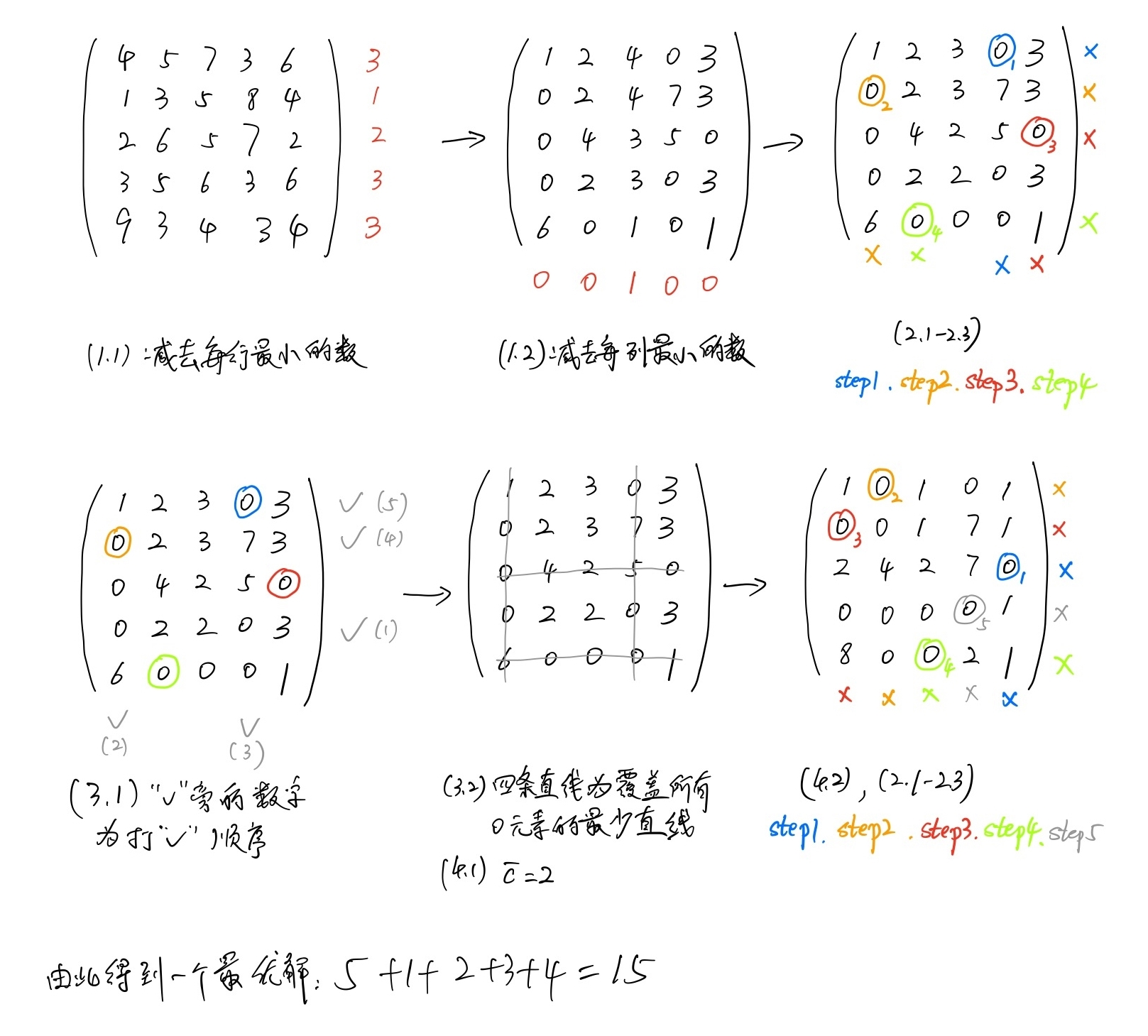Jarvis' Blog (总有美丽的风景让人流连) 总有美丽的风景让人流连

# 匈牙利算法 (Hungarian Algorithm)

2022-05-23
Jarvis
Post

扫地 浇花 擦窗户

## 2. 匈牙利算法

1. 效益矩阵初始化
• (1.1) 将矩阵 $$\mathbf{C}$$ 每行减去该行的最小元素, 得新矩阵 $$\mathbf{C}'$$;
• (1.2) 将矩阵 $$\mathbf{C}'$$ 每列减去该列的最小元素, 得新矩阵 $$\mathbf{C}''$$, 以下操作对 $$\mathbf{C}''$$ 进行;
2. 对当前效益矩阵求可行解
• (2.1) 从含零最少的行(或列)开始, 圈出矩阵中的一个 “0”, 并划去其所在的行和列;
• (2.2) 重复步骤 (2.1) 直至矩阵中所有的 “0” 均被划去或圈出为止;
• (2.3) 若已圈出 $$n$$ 个 “0”, 它们即对应一最优解, 如不然转步骤 3.
3. 求覆盖当前效益矩阵中所有 “0” 的最少条数直线
• (3.1) 对没有圈出 “0” 的行打 $$\checkmark$$, 对打 $$\checkmark$$ 行上所有 “0” 所在的列打 $$\checkmark$$, 再对打 $$\checkmark$$ 的列上所有有 “0” 被圈出的行打 $$\checkmark$$, 直至打不出 $$\checkmark$$ 为止;
• (3.2) 将没有打 $$\checkmark$$ 的行和打 $$\checkmark$$ 的列划上直线, 这些直线即为所求.
4. 调整效益矩阵
• (4.1) 找出没有被直线覆盖的所有元素中最小的数 $$\bar{c}$$;
• (4.2) 将打 $$\checkmark$$ 的行上每个元素减去 $$\bar{c}$$, 打 $$\checkmark$$ 的列上每个元素加上 $$\bar{c}$$, 得到新的效益矩阵. 返回步骤 2.

## 3. 一个稍复杂的例子Content